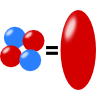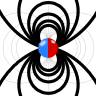# Multipole Moments of the Electric Field

The multipole moments of a charge distribution $$\rho\left(\mathbf{r}\right)$$ arise naturally if we try to solve Poisson's equation for the electrostatic potential, $$\Delta\phi\left(\mathbf{r}\right)= -\rho\left(\mathbf{r}\right)/\varepsilon_{0}$$:
The Green's function of the Laplace operator obeying $$\Delta_{\mathbf{r}}G\left(\mathbf{r},\mathbf{r}^{\prime}\right) = \delta\left(\mathbf{r}-\mathbf{r}^{\prime}\right)$$, is given by $$G\left(\mathbf{r},\mathbf{r}^{\prime}\right) = -1/4\pi\left|\mathbf{r}-\mathbf{r}^{\prime}\right|$$, which provides us the well-known $\phi\left(\mathbf{r}\right)=\frac{1}{4\pi\varepsilon_{0}}\int\frac{\rho\left(\mathbf{r}^{\prime}\right)}{\left|\mathbf{r}-\mathbf{r}^{\prime}\right|}dV\ .$The Green's function can now be expanded in a Taylor series around $$\mathbf{r}^{\prime}=0$$ yielding (see also in the solution of "The Electric Field of a Dipole")$\begin{eqnarray*}\phi\left(\mathbf{r}\right) & = & \frac{1}{4\pi\varepsilon_{0}}\left\{\int\frac{\rho\left(\mathbf{r}^{\prime}\right)}{\left|\mathbf{r}\right|}dV^{\prime}+\int\frac{\mathbf{r}\cdot\mathbf{r}^{\prime}\rho\left(\mathbf{r}^{\prime}\right)}{\left|\mathbf{r}\right|^{3}}dV^{\prime}+\dots\right\}\\&=& \frac{1}{4\pi\varepsilon_{0}}\left\{\frac{Q}{r}+\frac{\mathbf{p}\cdot\mathbf{r}}{r^{3}}+\dots\right\}\end{eqnarray*}$with $$Q=\int\rho\left(\mathbf{r}^{\prime}\right)dV^{\prime}$$ as the total charge, the dipole moment $$\mathbf{p}=\int\mathbf{r}^{\prime}\rho\left(\mathbf{r}^{\prime}\right)dV^{\prime}$$ and $$r=\left|\mathbf{r}\right|$$. You can see that the moments we already know are just the beginning of a fundamental representation of the electrostatic potential. In the following problems we shall make ourselfs familier with the multipole moments. Enjoy!

## The Quadrupole Moment of a Homogeneously Charged EllipsoidThe quadrupole moments of a homogeneously charged ellipsoid are calculated. The result will help us to understand how and why the quadrupole moments of an ellipsoidal charge distribution changes if it gets into an excited state.

## A Simple Model for Atomic Nuclei: The Deformed Sphere and its Multipole MomentsWe will calculate the first three multipole moments of a deformed charged sphere. Such a charge distribution is often used to model nuclei and understand their stability. The rotational symmetry of the problem will ease our computations significantly.

## The Electric Field of a DipoleThe electric field of a dipole can be seen as the result of two charges approaching each other. Learn in this problem how to use the Taylor expansion of $$1/\left|\mathbf{r}-\mathbf{r}^{\prime}\right|$$ to calculate this field. Find out how this series expansion yields multipole moments, a very powerful description of the electric field.

## Multipole Moments in Rotational SymmetryIn rotational symmetry, the multipole moments of a charge distribution are described by just one component per order $$l$$. This is a drastic difference to the usual $$2l+1$$ independent components. Get to know this simplifications with two examples and derive it for any charge distribution with rotational symmetry!